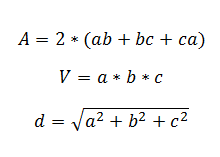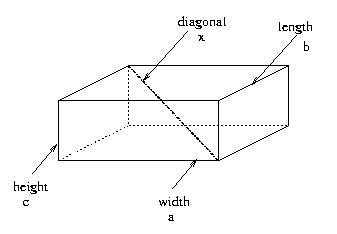# Right Parallelepiped

This CalcTown calculator calculates the surface area, volume and body diagonal length of a right parallelepiped.

#### Result

m2
m3
mWhere,

a = side 1 of the parallelepiped

b = side 2 of the parallelepiped

c = side 3 of the parallelepiped

d = length of body diagonal

A = surface area of the parallelepiped

V = volume of the parallelepiped